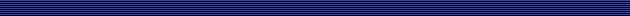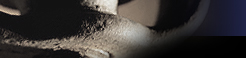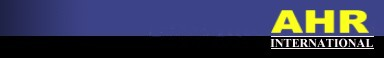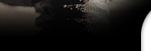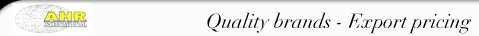Stock search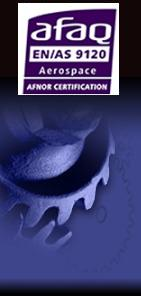### TASC DRIVES bearing references

Please find below the current bearing references we have for TASC DRIVES. If you don't see the bearing reference you are seeking for this OEM then please do not hesitate to contact us for prompt support.

 60000 61100 62501 BE600000 BE600000 BE600000 BE600660 BE611000 BE611080 BE611250 BE611250 BE611340 BE611370 BE611390 BE611470 BE611480 BE611490 BE625260 BE625270 BE625280 BE625290 BE625310 BE625411 BE625461 BE625461 BE625481 BE625491 BE643061 BE643071 BE643091 BE643101